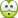# Projectile motion -- throwing an object vertically

hescot2
Homework Statement:
.
Relevant Equations:
.
I don't need to know how to solve this but I was just looking for the answer. Can anyone help?

A man is standing at a launch site. The rocket doesn't liftoff. Frustrated s/he throws it straight up with a speed of 12.42 m/s. It is caught on the way down at a point 5.0 meters above where it was thrown, by a fellow rocketeer on the second floor, who doesn't want to see any harm come ot this wonderful rocket. The first man wants to figure out how fast the rocket was going when it was caught. Again thanks for all your help, really rusty with this right now.

Homework Helper
Gold Member
2022 Award
Homework Statement:: .
Relevant Equations:: .

I don't need to know how to solve this but I was just looking for the answer.
So it isn't homework?

hescot2
no. I am too old for homework. just trying to solve with friends for a game

Is it ok to ask here?

Mentor
Homework Statement:: .
Relevant Equations:: .

I don't need to know how to solve this but I was just looking for the answer. Can anyone help?

A man is standing at a launch site. The rocket doesn't liftoff. Frustrated s/he throws it straight up with a speed of 12.42 m/s. It is caught on the way down at a point 5.0 meters above where it was thrown, by a fellow rocketeer on the second floor, who doesn't want to see any harm come ot this wonderful rocket. The first man wants to figure out how fast the rocket was going when it was caught. Again thanks for all your help, really rusty with this right now.
no. I am too old for homework. just trying to solve with friends for a game

Is it ok to ask here?

Welcome to the PF. No, it is against the rules to post schoolwork type questions and not show any work. That's not how the PF works. Here is a link that helps to explain the reasons for us treating all homework and schoolwork-type threads this way:

Hope that makes sense. You will probably still find the PF useful as a learning tool, but we won't solve problems like this for you. We can give you a couple hints and point you to the equations that you will need to understand and use to solve this problem, though. Let us know if you want the hints and pointers to the equations to use.

hescot2
Sorry! Are they equations that a 60 year old could do?? I am so far from doing anything like this. I appreciate any help that could streer me in the right direction.

One of the equations you can use when you're dealing with constant acceleration is$${v_y}^2 = {u_y}^2 + 2a_y \Delta y$$where ##u_y = 12.42 \text{ms}^{-1}##, ##a_y = -g##, ##\Delta y = 5.0 \text{m}##. Hopefully you see that there are two solutions for ##v_y## of equal magnitude, and you're interested in the solution at time ##t_2##, with ##t_2 > t_1##, where ##v_y < 0##.

•berkeman
Mentor
And ##g## it the acceleration due to gravity, which is ##9.8\frac{m}{s^s}## for this problem.

Try working on that equation and post your work, and we can try to help you through it. Think of it as a learning adventure...•etotheipi
Homework Helper
2022 Award
I've got almost a decade on you, so yes we do. Did you take physics in school?
I see @etotheipi has used conservation of energy to supply you a good start.

•etotheipi
hescot2
thank you for the response but I understand none of that. I guess that I am just too old.sorry for bothering you and thank you for helping as much as you were allowed to! If you are ever interested in the game that this applies to,

https://www.geocaching.com/geocache/GCKNKX

hescot2
someone in our group came up with 9.365. Are you allowed to verify that?

someone in our group came up with 9.365. Are you allowed to verify that?

Sorry, it's wrong.

hescot2
ugg. Thanks.

Homework Helper
Gold Member
2022 Award
ugg. Thanks.
Maybe you could get whoever came up with that number to explain the reasoning and post that. Then we can progress.

•hutchphd
hescot2

Homework Helper
Gold Member
2022 Award
Yes.

hescot2
THANK YOU!

•hutchphd
Mentor
Do we win a prize?

One of the equations you can use when you're dealing with constant acceleration is$${v_y}^2 = {u_y}^2 + 2a_y \Delta y$$
Personally, that is the one SUVAT equation that I have never bothered to memorize. But divide by two and multiply by m and you get the equation that I use all the time:$$\frac{1}{2}mv_y^2 = \frac{1}{2}mu_y^2 + ma_y\Delta y$$Which of course is an assertion about kinetic energy after an acceleration in terms of kinetic energy before the acceleration plus work done by the acceleration.
•Sure that works! You can also get it with $$\Delta y = \frac{1}{2}(u_y + v_y)t = \frac{1}{2} (u_y + v_y) \frac{(v_y - u_y)}{a_y} = \frac{v_y^2 - u_y^2}{2a_y}$$
•